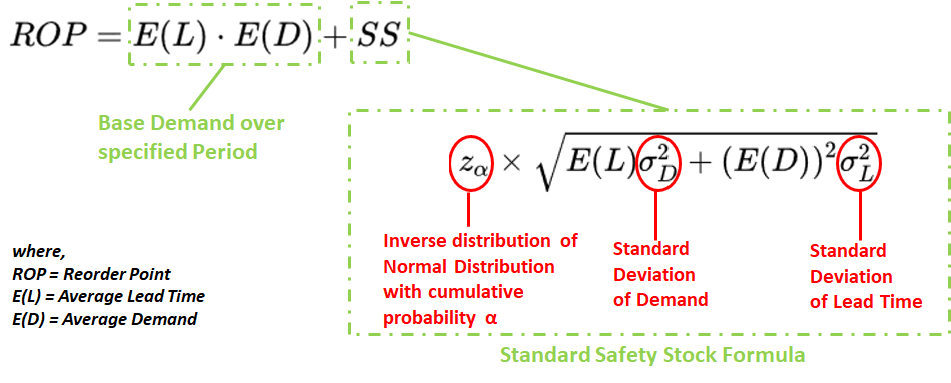Search

# From Supply Chain Inventory to Rainfall to Financial Risk: When Normal(distribution) is NOT the Norm

Updated: Mar 5, 2021Most of us have heard of the famous Normal Distribution (a.k.a Gaussian Distribution, a.k.a “Bell’s Curve). What some of us may have forgotten, is that Normal Distribution’s popularity is powered by the Central Limit Theorem, definition below:

“Central Limit Theorem states that the sampling distribution of the sample means approaches a Gaussian Normal distribution as the sample size gets larger — no matter what the shape of the population distribution.”

In plain English, CLT implies that probabilistic and statistical methods that work for normal distributions can be applicable to many problems involving other types of non-normal distributions.

The only caveat(s)? Your sample data must meet all 3 conditions below:

1. Randomized Sampling: The data must be sampled randomly. Is one of the good sampling methodologies discussed in the chapter “Sampling and Data” being used?

2. Independence: The sample values must be independent of each other. This means that the occurrence of one event has no influence on the next event.

3. Sample Size: The sample size must be sufficiently large.

#2 can indeed be tested through various statistical techniques, one of which being ANOVA (Analysis of Variance).

#3 is where it gets really vague. What defines “sufficiently large”? From prevailing literature, it appears a “sufficiently large” range can stretch from 30 to Infinity.

Yet, despite meeting Conditions 1 and 2, and Condition 3 (with very large sample sizes, certainly not infinity!) non-normal behaviour is exhibited through a wide range of physical and social phenomena. Some examples are listed below:

• Geology: Concentration of Elements in Earth’s core

• Medicine: Survivor times after cancer diagnosis

• Ecology: Inheritance of fruit and petal sizes

• Environment: Amount of Rainfall

• Linguistics: Length of spoken words in phone conversations

• Business: Call Centre Service Times

Incidentally, because Lognormal distribution describes all the above phenomena very well, let’s use this as an illustrative comparison to Normal Distribution.

Below is a graphical comparison of a Normal Distribution (in yellow square), compared with Lognormal Distributions:

• The Normal Distribution has a mean of 100 and standard deviation of 20

• The other curves, which are lognormal, share a mean of 100. However, their standard deviations are variedGraphical comparison of a Normal Distribution (in yellow square), compared with Lognormal Distributions

From the chart, we can make a few observations:

1. The more Coefficient-of-Variation (COV) approaches 0, the more a Lognormal Distribution resembles a Normal Distribution.

2. A Lognormal Distribution with a COV of just 0.2 already differs significantly from a visual standpoint

3. Holding only the mean constant and changing only standard deviations, a Normal Distribution overestimates values that are most likely the occur (as measured by the peak points on the curves – which have the highest likelihood of occurrence)

So what does all of these have to do with Supply Chain Inventory Planning?

Here is the inventory planning (Re-Order Point) equation still largely in use by most organisations today:We can clearly see that Normal Distribution assumptions are embedded in the common Inventory Planning equations, because of:

1. Standard Deviations

2. Zα is meant for standard deviations Eg. Zα = 1.645 when cumulative probability of a normal distribution is 95%

BUT what if Demand does NOT follow a Normal Distribution, but Lognormal (Like many things in nature)? What will it mean for Inventory Planning?

• Firstly, there is a basic reason why most SKUs probably do not follow a Normal Distribution: Going by the Pareto Rule, around only around 20% of SKUs would generate 80% of demand (Low Mix/High Volume) and are more likely to demonstrate stable demand Hence, remaining 80% of SKUs would have significantly less demand (High Mix/Low Volume) and are more likely to demonstrate volatile demand

• Secondly, estimated demand can vary significantly between the 2 distributions (with the same Mean and Standard Deviation)

The below table shows projected stocks required to cover a client’s peak demand, at a desired confidence level %. Both distributions assume a mean of 100, and standard deviation of 250 (volatile demand)As one can see, Normal Distribution tends to over-estimate the required stocks until around 98% confidence level %. For context, most service level agreements stipulate a minimum service level of 95%.

Then, why was there such a reliance on Normal Distribution?

Mainly due to 3 reasons:

1. Normal distribution is considerably easier to compute. In a bygone era of low processing power, usage of normal distribution could quickly reap significant benefits delivered by statistical techniques

2. Normal distributions are more “intuitive” to understand due to inherent symmetry

3. History of distributions: Normal distributions have been applied twice as long as it’s log-normal sister distribution (and even longer relative to other distributions like Weibull)

So how can Supply Chain Organisations choose the appropriate Distribution for their supply chain demand - not only for today, but ongoingly?

• In addition to lognormal distribution, consider also other statistical distributions such as Rayleigh, Weibull, Gamma, etc. Remember that Lognormal is just used as an example alternative to Normal distribution in this article

• Consider Multi-Echelon Inventory Optimization (MEIO), and segmentize SKUs according to different criteria such as demand volatility, volume, profit margin, et cetera. To each SKU segmentation, deploy a different forecasting and inventory policy.

• Given big leaps in computing power, consider leveraging software and smart analytics tools that will dynamically advise on historical trends and supplement MEIO (it could recommend an SKU to be moved to another segment, with an ongoing change in forecasting and inventory policy) Gartner’s 2018 Quadrant for Supply Chain Planning software (which includes inventory planning) is as below. Highlighted in red is the Leaders’ Quadrant indicating High execution ability and High completeness of vision.• On top of analytical tools and SKU segmentation, set up a rigorous periodic process to review exceptional SKUs within SKU segments (which again, can be supplemented by software and tools)

Johnson Chua - Forizons Hong Kong Director